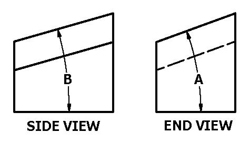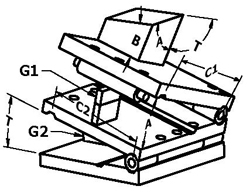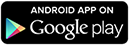Suburban Tool, Inc.
 HOW TO USE A COMPOUND SINE PLATE
 When setting compound angles, it is necessary to compensate for the first angle set in order to correctly set the second angle. Refer to the following drawings and procedures.  The compensation will be calculated for you.Where: Angle A = First desired workpiece angle. Angle B = Second desired workpiece angle. Angle T = True angle setting required to get angle B. To set the angles on a compound sine plate, you must first determine the top plate center distance of the device (C1), the bottom plate center distance (C2), the workpiece angles you wish to set (A & B) and whether the angles are in degrees-minutes-seconds or decimal degrees.  Next, enter that information in the appropriate input areas below.  Use a decimal point for the separator, whether the angle is in degrees-minutes-seconds or decimal degrees.  Hit the 'Calculate' button and then assemble the stacks of gage blocks (G1 & G2) to equal the size that is returned.  The units of the stack will match the units of the center distance (i.e., If you enter the center distance as 5 for 5 inches, the gage block stack will also be in inches.).  Set the upper section of your compound sine plate, using the gage block stack G1.  This sets your required angle A.  Set the lower section of your compound sine plate, using the gage block stack G2.  This sets the true angle T.  Results: The compound sine plate is now set to obtain the desired angles A & B in their respective planes on the workplace.To compensate for the compound angle:Tan(True Angle T) = Tan(B) x Cos(A)
 Top Plate Center Distance  [ 'C1' in drawing above ] Workpiece Angle 'A' Type Degrees Minutes Seconds  [ DD.MM.SS ]     Decimal Degrees  [ DD.DDDD ] Workpiece Angle To Set  [ 'A' in drawing above (0 to 50 degrees) ]
 Bottom Plate Center Distance   [ 'C2' in drawing above ] Workpiece Angle 'B' Type Degrees Minutes Seconds  [ DD.MM.SS ]     Decimal Degrees  [ DD.DDDD ] Workpiece Angle To Set   [ 'B' in drawing above (0 to 50 degrees) ]
 Download the Android version of our our new"HOW TO USE A SINE PLATE" appatBy using this site you agree to our Privacy &Security Policy, Sales Policy Website Terms of Use Policy

 4141 N. Atlantic Boulevard, Auburn Hills, Michigan 48326 Phone: (248)391-7800 Fax: (248)391-7462 E-mail: tools@subtool.com Copyright © 2001-2018 - Suburban Tool, Inc. All rights reserved. All trademarks and logos shown are the property of their respective owners.